15.3 随机森林的细节¶

weiya 注：Recall

• 对于分类，$m$ 的默认值为 $\lfloor \sqrt p \rfloor$，且最小的结点数为 1．
• 对于回归，$m$ 的默认值为 $\lfloor p/3\rfloor$，且最小的结点数为 5．

Recall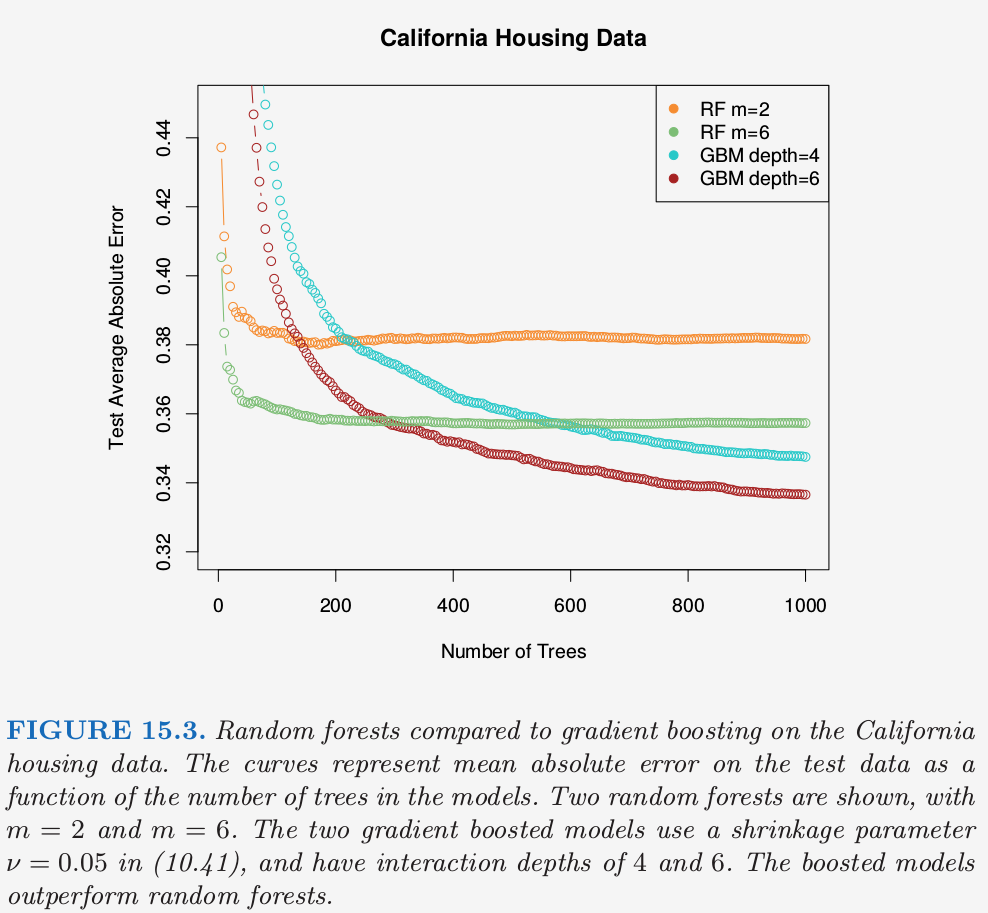集外样本¶

OOB 误差估计几乎等价于通过 $N$ 折交叉验证得到的估计；见练习 15.2．因此与许多其它的非线性估计量不同，随机森林可以逐步拟合，同时交叉验证也将沿着拟合进度逐步进行．一旦OOB误差稳定，训练可以终止．

weiya 注：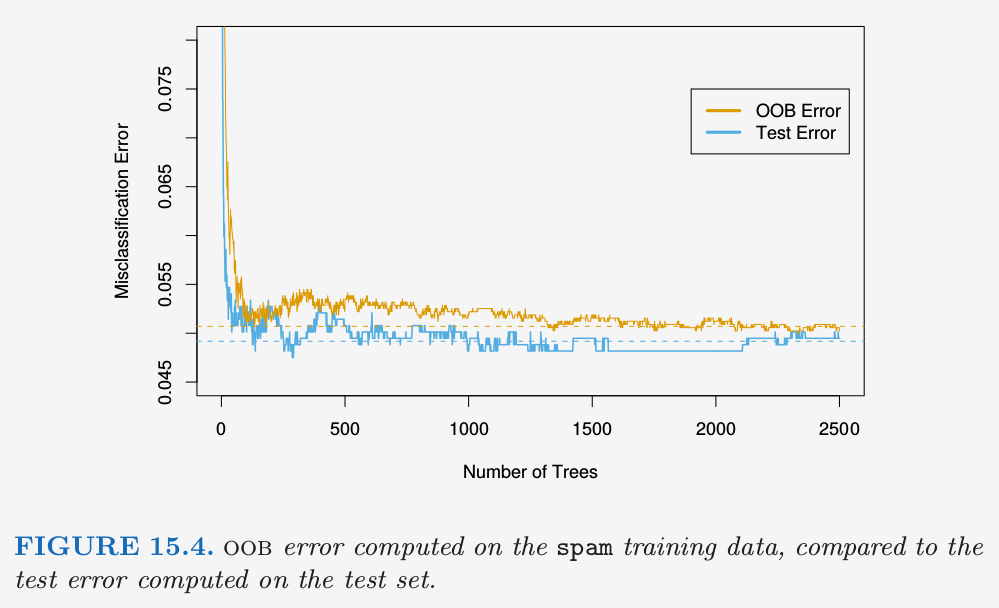变量重要性¶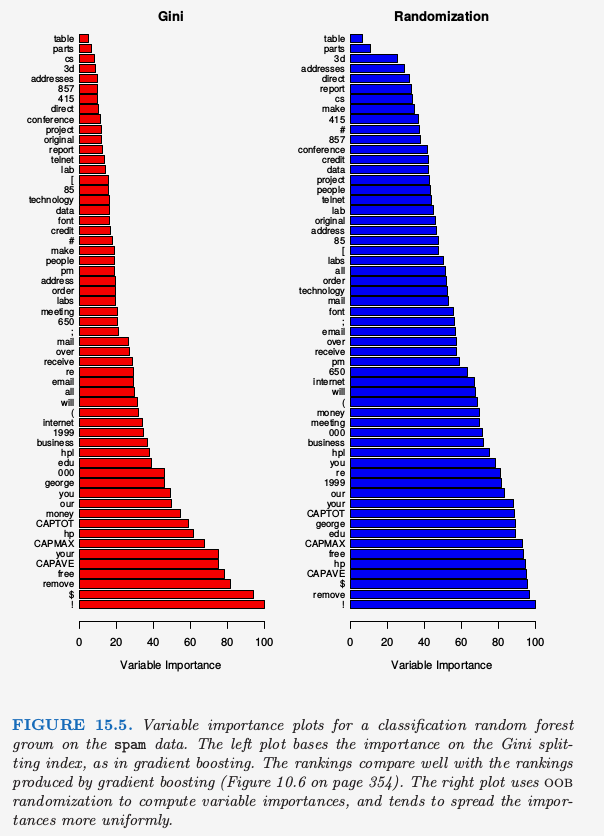Recall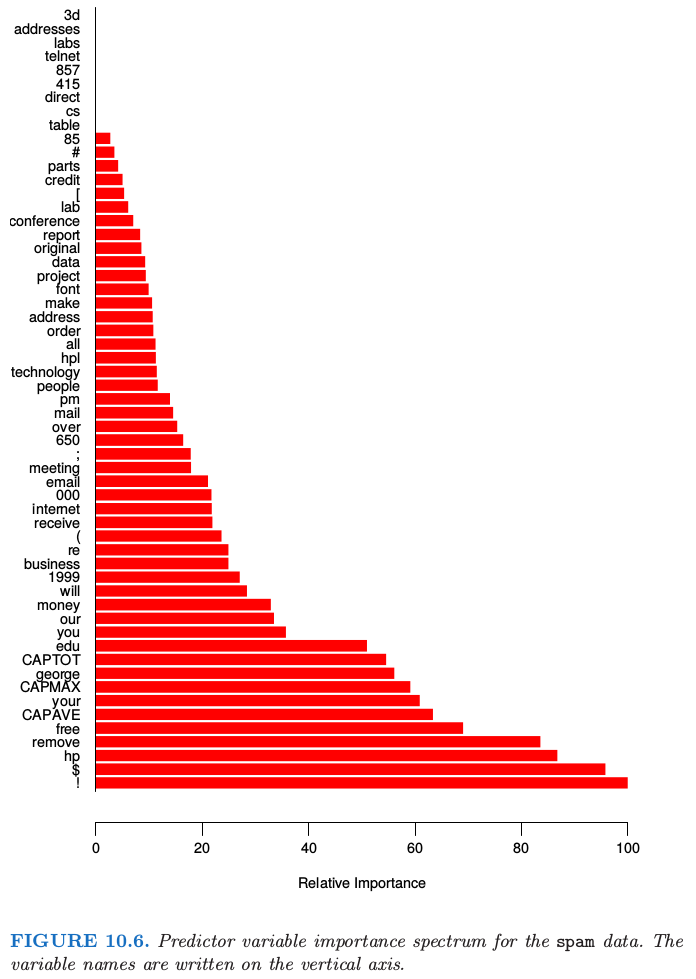Boosting 完全忽略了一些变量，而随机森林不会．备选的分离变量选择增加了任意单个变量被包含进随机森林的概率，而 boosting 中没有这种选择．

weiya 注：Permutation Importance

邻近图¶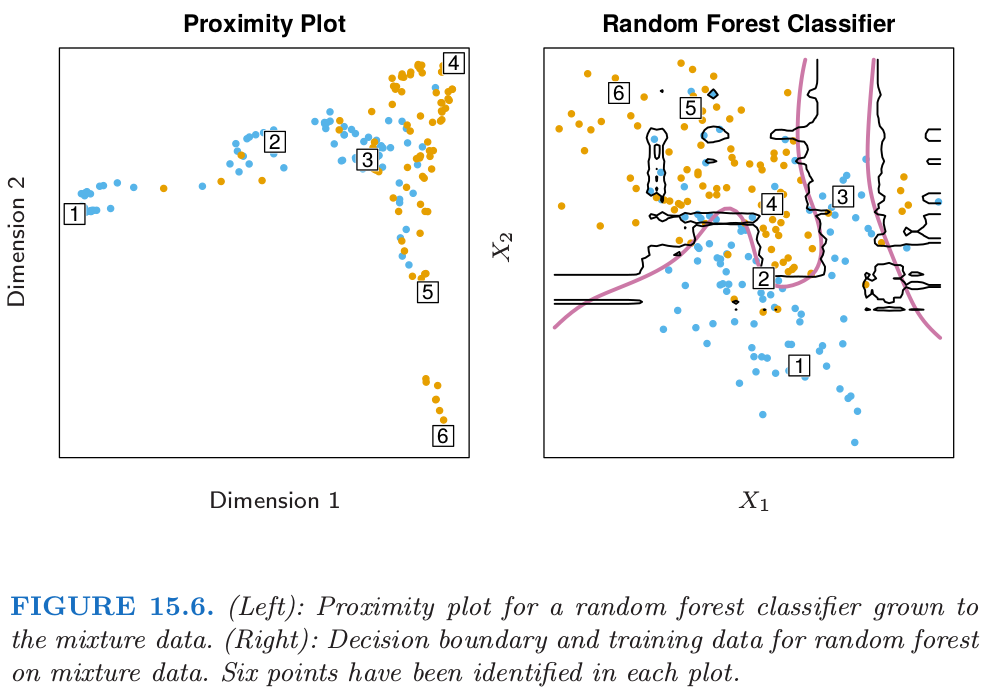weiya 注：

随机森林和过拟合¶

weiya 注：Ex. 15.3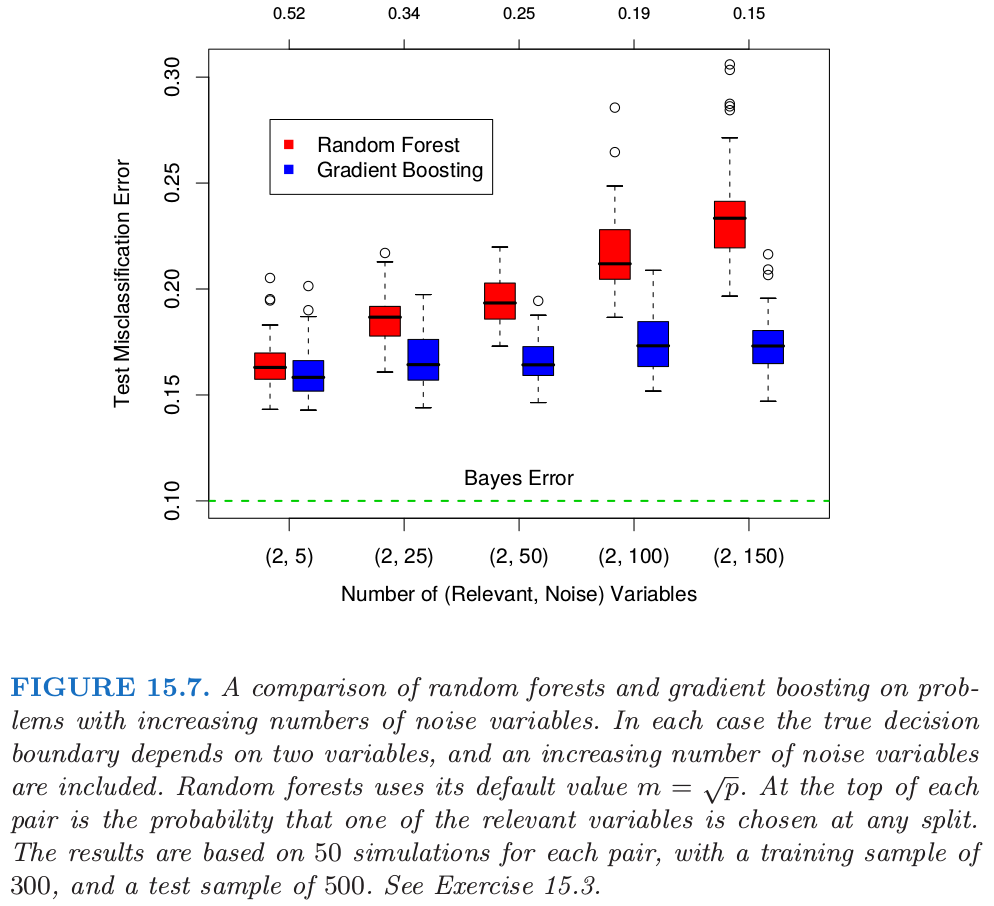weiya 注:

f(n1, n2; m = round(Int, sqrt(n1 + n2))) = sum([binomial(n1, i) * binomial(n2,m-i ) for i=1:min(n1, m)]) / binomial(n1+n2, m)
f(6, 100) #0.4565650244108061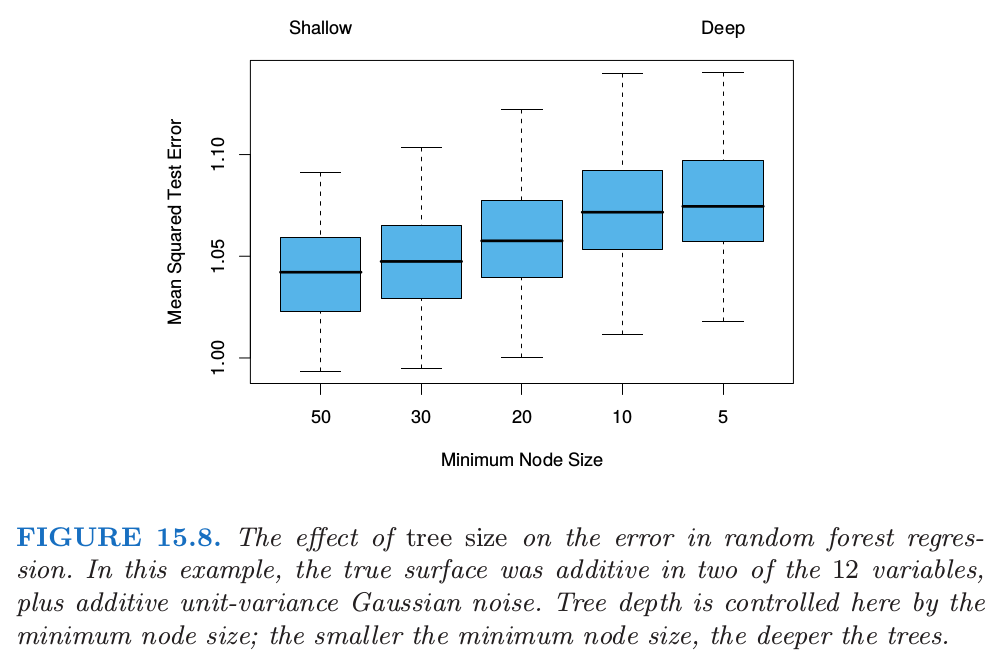1. Segal, M. (2004). Machine learning benchmarks and random forest regression, Technical report, eScholarship Repository, University of California. http://repositories.edlib.org/cbmb/bench rf regn .